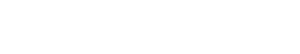# Geometric Distribution

The geometric distribution is used to calculate the probability that a given word will appear in a document. For example, if we have a corpus of 100 documents and we want to know the probability that the word “cat” will appear in one of them, we would use the geometric distribution.

The geometric distribution is also used outside of the text analytics industry, but it has a different meaning. In statistics, the geometric distribution is used to calculate the probability of success in a Bernoulli trial. For example, if we have a coin that we flip 100 times, and we want to know the probability that it will come up heads 50 times, we would use the geometric distribution.

The formula for the probability mass function of the geometric distribution is:

p(x) = (1-p)^(x-1) * p

Where:

x is the number of failures

These are some synonyms or closely related terms to geometric distribution:

• Negative binomial distribution
• Poisson distribution
• Pascal distribution
• waiting time distribution

The geometric distribution is often confused with the binomial distribution, but they are not the same. The binomial distribution is used to calculate the probability of a given number of successes in a fixed number of trials, while the geometric distribution is used to calculate the probability of a given number of failures before the first success.

## Use of the Geometric Distribution

The use of the geometric distribution in text analytics is to help understand the likelihood of how often a word or phrase is used. For example, if you are looking at a document and you see the word “apple” 50 times, you can use the geometric distribution to calculate the probability that the next time you see the word “apple” it will be the 51st time.Unlock the power of actionable insights with AI-based natural language processing.